• 数据缺失值补全方法sklearn.impute.SimpleImputer imp=SimpleImputer(missing_values=np.nan,strategy=’mean’) 创建该类的对象，missing_values,也就是缺失值是什么，一般情况下缺失值当然就是空值啦，也就是np....
• 起因非常复杂，我想装smar的包，然后报错impute包安装失败。然后试图利用install.packages("impute")安装，再次失败，报错：package ‘impute’ is not available (for R version 3.6.1) 【注意】我的R版本好像是...
起因非常复杂，我想装smar的包，然后报错impute包安装失败。然后试图利用install.packages("impute")安装，再次失败，报错：package ‘impute’ is not available (for R version 3.6.1)
【注意】我的R版本好像是3.6.4.
目前在网上找到的方法大多是：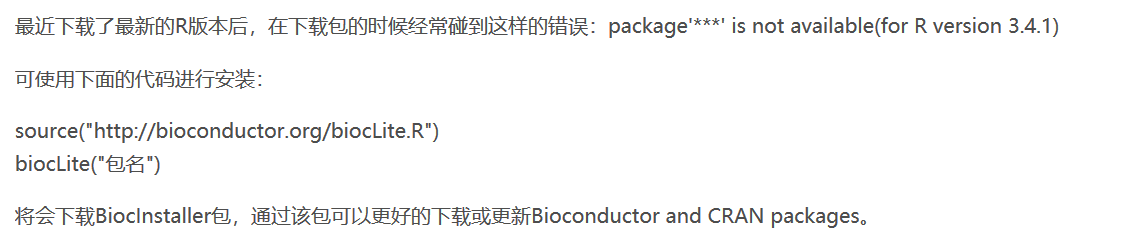但是我使用的时候发现，依旧报错。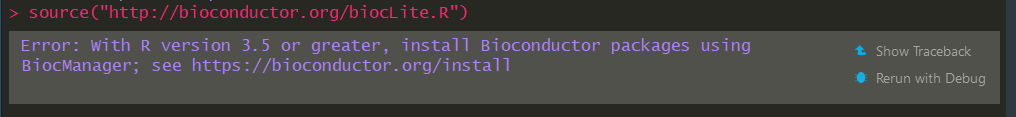然后在biocunductor的网站上找到了impute的新安装方式（链接：bioconductor）： 发现这段代码主要针对3.6以上的R版本
if (!requireNamespace("BiocManager", quietly = TRUE))
install.packages("BiocManager")

BiocManager::install("impute")

使用之后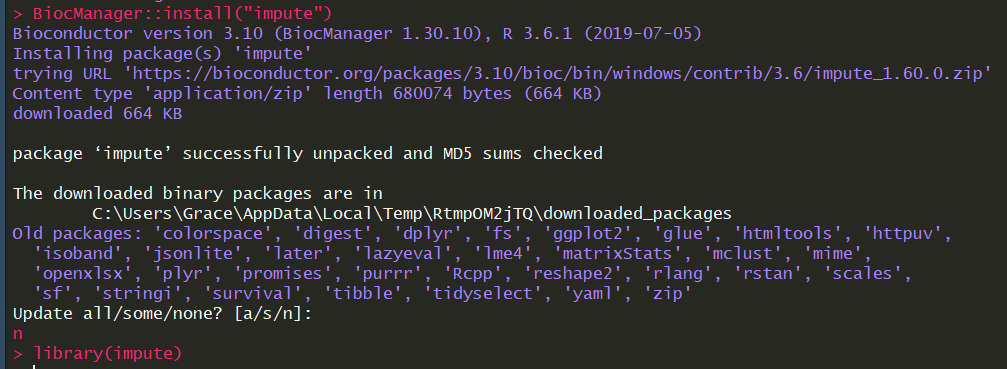注意，如果时间紧张，在update all/some/none那里，最好选n，因为update all真的要好久，久到我读了27页的垃圾书。（不是所有书的问题，是我选的那本书的问题。被名气欺骗了，难过。）
Ref: http://www.bioconductor.org/packages/release/bioc/html/impute.html
展开全文• AIS-impute-master_softimpute_ais23.com_matrixcompletion_ais_bendy1r_源码.rar.rar
• Sklearn 有专门处理缺失值的模块 sklearn.impute.SimpleImputer，本文将探究如何用 Sklearn 中的预处理模块中的 Impute.SimpleImputer 处理缺失值。 一、模块介绍 官网详解在 part 6.4 Imputation of missing values...
Sklearn 有专门处理缺失值的模块 sklearn.impute.SimpleImputer，本文将探究如何用 Sklearn 中的预处理模块中的 Impute.SimpleImputer 处理缺失值。
一、模块介绍 官网详解在 part 6.4 Imputation of missing values：https://scikit-learn.org/stable/modules/impute.html
它包含四个重要参数：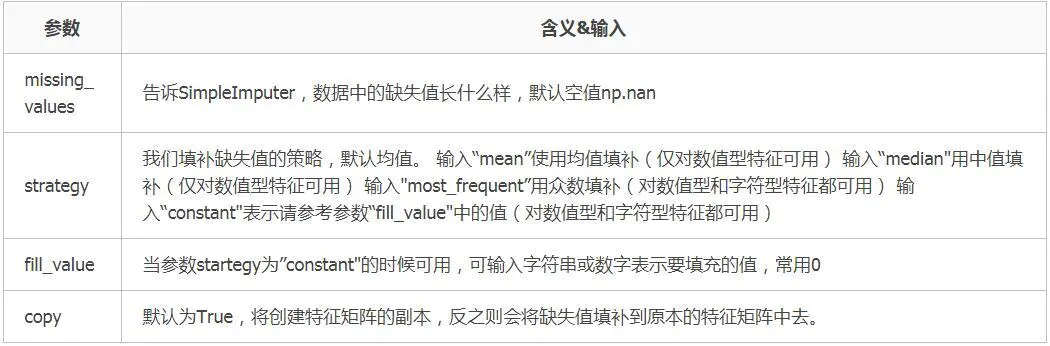二、示例应用 接下来将用实例来讲解如何使用填补缺失值：
1）数据来源及数据基本信息
>>> from sklearn.impute import SimpleImputer
>>> import pandas as pd
>>> import numpy as np
>>> data.info()
<class 'pandas.core.frame.DataFrame'>
Int64Index: 891 entries, 1 to 891
Data columns (total 11 columns):
Survived    891 non-null int64
Pclass      891 non-null int64
Name        891 non-null object
Sex         891 non-null object
Age         714 non-null float64
SibSp       891 non-null int64
Parch       891 non-null int64
Ticket      891 non-null object
Fare        891 non-null float64
Cabin       204 non-null object
Embarked    889 non-null object
dtypes: float64(2), int64(4), object(5)
memory usage: 83.5+ KB
>>> data.shape
(891, 11)

数据概要：实例使用的是泰坦尼克号数据集，查看数据集基本信息，发现Age、Cabin、Embarkde这三个字段均有数据缺失。其中Cabin缺失687条，Age缺失177条，Embarked缺少2条数据。下面示例应用将以填充数据集的[Age]字段为例。
2）缺失值处理应用 1、均值填充
age=data['Age'].values.reshape(-1,1)  #取出缺失值所在列的数值，sklearn当中特征矩阵必须是二维才能传入 使用reshape(-1,1)升维

from sklearn.impute import SimpleImputer #导入模块
imp_mean=SimpleImputer(missing_values=np.nan,strategy='mean')  #实例化，均值填充
imp_mean=imp_mean.fit_transform(age)     #fit_transform一步完成调取结果

data['Age']=imp_mean       #填充好的数据传回到 data['Age']列

data['Age'].isnull().sum() #检验是否还有空值，为0即说明空值均已被填充

2、中值填充
age=data['Age'].values.reshape(-1,1)         #取出缺失值所在列的数值，sklearn当中特征矩阵必须是二维才能传入 使用reshape(-1,1)升维

from sklearn.impute import SimpleImputer     #导入模块
imp_median=SimpleImputer(missing_values=np.nan,strategy='median')    #实例化，中值填充
imp_median=imp_median.fit_transform(age)     #fit_transform一步完成调取结果
data['Age']=imp_median       #填充好的数据传回到 data['Age']列
data['Age'].isnull().sum()   #检验是否还有空值，为0即说明空值均已被填充

3、众数填充
age=data['Age'].values.reshape(-1,1)  #取出缺失值所在列的数值，sklearn当中特征矩阵必须是二维才能传入 使用reshape(-1,1)升维

from sklearn.impute import SimpleImputer #导入模块
imp_most_frequent=SimpleImputer(missing_values=np.nan,strategy='most_frequent')  #实例化，众数填充
imp_most_frequent=imp_most_frequent.fit_transform(age)     #fit_transform一步完成调取结果
data['Age']=imp_most_frequent       #填充好的数据传回到 data['Age']列
data['Age'].isnull().sum()          #检验是否还有空值，为0即说明空值均已被填充

4、常数填充（填充0，填充1等常数）
age=data['Age'].values.reshape(-1,1)  #取出缺失值所在列的数值，sklearn当中特征矩阵必须是二维才能传入 使用reshape(-1,1)升维

from sklearn.impute import SimpleImputer       #导入模块
imp_0=SimpleImputer(missing_values=np.nan,strategy='constant',fill_value=0)  #实例化，填充常数0，填充常数需strategy与fill_value一同使用
imp_0=imp_0.fit_transform(age)     #fit_transform一步完成调取结果
data['Age']=imp_0                   #填充好的数据传回到 data['Age']列
data['Age'].isnull().sum()          #检验是否还有空值，为0即说明空值均已被填充

转自：https://www.jianshu.com/p/2c59147e1df1
展开全文• no moudle named sklearn-impute一、原因分析二、处理过程处理命令 一、原因分析 原因：impute是sklearn里面的一个包，没有这个包的原因自然就是sklearn的版本太低，需要更新出来这个包的版本，这个时候可以先查看...


no moudle named sklearn-impute
一、原因分析二、处理过程处理命令

一、原因分析
原因：impute是sklearn里面的一个包，没有这个包的原因自然就是sklearn的版本太低，需要更新出来这个包的版本，这个时候可以先查看自己的版本，在pycharm里面，终端输入pip list，查看自己的sklearn的版本。如果是.19的话，其实是没有这个包的。
二、处理过程
直接安装的话，会出现已经存在sklearn 的错误提示。此时要么删除之前的版本，要么就是忽略这个之前的包 直接安装覆盖
处理命令
pip install --ignore-installed  scikit-learn

我是这么处理的，时候pip list查看之后，就会出现sklearn的版本已经是.22的了。自然就不会出现错误了。
展开全文tensorflow
• Impute 1、数据无量纲化 ​ 在机器学习算法实践中，我们往往有着将不同规格的数据转换到同一规格，或不同分布的数据转换到某个特定分布的需求，这种需求统称为将数据“无量纲化”。譬如梯度和矩阵为核心的算法中，...
数据预处理1 Preprocessing & Impute
1、数据无量纲化
​ 在机器学习算法实践中，我们往往有着将不同规格的数据转换到同一规格，或不同分布的数据转换到某个特定分布的需求，这种需求统称为将数据“无量纲化”。譬如梯度和矩阵为核心的算法中，譬如逻辑回归，支持向量机，神经网络，无量纲化可以加快求解速度；而在距离类模型，譬如K近邻，K-Means聚类中，无量纲化可以帮我们提升模型精度，避免某一个取值范围特别大的特征对距离计算造成影响。
​ 数据的无量纲化可以是线性的，也可以是非线性的。线性的无量纲化包括中心化（Zero-centered或者Mean-subtraction）处理和缩放处理（Scale）。中心化的本质是让所有记录减去一个固定值，即让数据样本数据平移到某个位置。缩放的本质是通过除以一个固定值，将数据固定在某个范围之中，取对数也算是一种缩放处理。
​ 当数据(x)按照最小值中心化后，再按极差（最大值 - 最小值）缩放，数据移动了最小值个单位，并且会被收敛到[0,1]之间，而这个过程，就叫做数据归一化(Normalization，又称Min-Max Scaling)。注意，Normalization是归一化，不是正则化，真正的正则化是regularization，不是数据预处理的一种手段。归一化之后的数据服从正态分布，公式如下：​ 当数据(x)按均值(μ)中心化后，再按标准差(σ)缩放，数据就会服从为均值为0，方差为1的正态分布（即标准正态分布），而这个过程，就叫做数据标准化(Standardization，又称Z-score normalization)，公式如下：​ 大多数机器学习算法中，会选择StandardScaler来进行特征缩放，因为MinMaxScaler对异常值非常敏感。在PCA，聚类，逻辑回归，支持向量机，神经网络这些算法中，StandardScaler往往是最好的选择。
2、缺失值处理
​ 机器学习和数据挖掘中所使用的数据，永远不可能是完美的。很多特征，对于分析和建模来说意义非凡，但对于实际收集数据的人却不是如此，因此数据挖掘之中，常常会有重要的字段缺失值很多，但又不能舍弃字段的情况。因此，数据预处理中非常重要的一项就是处理缺失值。
import pandas as pd
week 3 Preprocessing\Narrativedata.csv",index_col=0)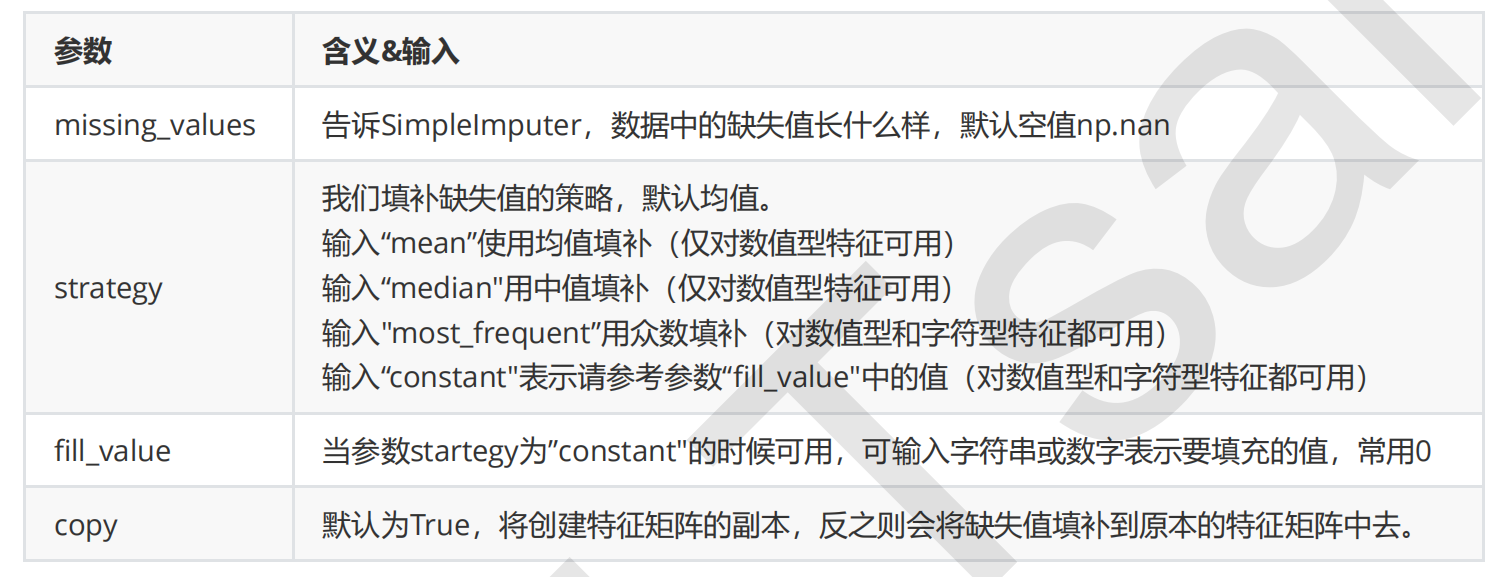data.info()
#填补年龄
Age = data.loc[:,"Age"].values.reshape(-1,1) #sklearn当中特征矩阵必须是二维
Age[:20]
from sklearn.impute import SimpleImputer
imp_mean = SimpleImputer() #实例化，默认均值填补
imp_median = SimpleImputer(strategy="median") #用中位数填补
imp_0 = SimpleImputer(strategy="constant",fill_value=0) #用0填补
imp_mean = imp_mean.fit_transform(Age) #fit_transform一步完成调取结果
imp_median = imp_median.fit_transform(Age)
imp_0 = imp_0.fit_transform(Age)
imp_mean[:20]
imp_median[:20]
imp_0[:20] #在这里我们使用中位数填补Age
data.loc[:,"Age"] = imp_median
data.info()
#使用众数填补Embarked
Embarked = data.loc[:,"Embarked"].values.reshape(-1,1)
imp_mode = SimpleImputer(strategy = "most_frequent")
data.loc[:,"Embarked"] = imp_mode.fit_transform(Embarked)
data.info()

#用Pandas和Numpy进行填补
import pandas as pd
Preprocessing\Narrativedata.csv",index_col=0)
data.loc[:,"Age"] = data.loc[:,"Age"].fillna(data.loc[:,"Age"].median())
#.fillna 在DataFrame里面直接进行填补
data.dropna(axis=0,inplace=True)
#.dropna(axis=0)删除所有有缺失值的行，.dropna(axis=1)删除所有有缺失值的列
#参数inplace，为True表示在原数据集上进行修改，为False表示生成一个复制对象，不修改原数据，默认False

3、 处理分类型特征：编码与哑变量
​ 在现实中，许多标签和特征在数据收集完毕的时候，都不是以数字来表现的。比如说，学历的取值可以是[“小 学”，“初中”，“高中”，“大学”]，付费方式可能包含[“支付宝”，“现金”，“微信”]等等。在这种情况下，为了让数据适应算法和库，我们必须将数据进行编码，即是说，将文字型数据转换为数值型。
3.1 preprocessing.LabelEncoder：标签专用，能够将分类转换为分类数值
from sklearn.preprocessing import LabelEncoder
y = data.iloc[:,-1] #要输入的是标签，不是特征矩阵，所以允许一维
le = LabelEncoder() #实例化
le = le.fit(y) #导入数据
label = le.transform(y)   #transform接口调取结果
le.classes_ #属性.classes_查看标签中究竟有多少类别
label #查看获取的结果label
le.fit_transform(y) #也可以直接fit_transform一步到位
le.inverse_transform(label) #使用inverse_transform可以逆转
data.iloc[:,-1] = label #让标签等于我们运行出来的结果
#如果不需要教学展示的话我会这么写：
from sklearn.preprocessing import LabelEncoder
data.iloc[:,-1] = LabelEncoder().fit_transform(data.iloc[:,-1])

3.2 preprocessing.OneHotEncoder：独热编码，创建哑变量
data.head()
from sklearn.preprocessing import OneHotEncoder
X = data.iloc[:,1:-1]
enc = OneHotEncoder(categories='auto').fit(X)
result = enc.transform(X).toarray()
result
#依然可以直接一步到位，但为了给大家展示模型属性，所以还是写成了三步
OneHotEncoder(categories='auto').fit_transform(X).toarray()
#依然可以还原
pd.DataFrame(enc.inverse_transform(result))
enc.get_feature_names()
result
result.shape
#axis=1,表示跨行进行合并，也就是将量表左右相连，如果是axis=0，就是将量表上下相连
newdata = pd.concat([data,pd.DataFrame(result)],axis=1)
newdata.drop(["Sex","Embarked"],axis=1,inplace=True)
newdata.columns ["Age","Survived","Female","Male","Embarked_C","Embarked_Q","Embarked_S"]



展开全文• AIS-impute-master_softimpute_ais23.com_matrixcompletion_ais_bendy1r_源码.zip
• 欢迎关注”生信修炼手册”!impute2是一款基因型填充软件，和其他软件相比，其填充的准确率最高，网址如下https://mathgen.stats.ox.ac.uk/impute/im...
• impute.knn(data.matrix(mat[, -1]), rowmax = thresh, colmax=0.5)\$data -> mat[, -1] 这样一个函数，运行到这里报错说找不到impute.knn 这个函数，在最新版本的R中也没有impute这个包，只有一个叫“imputeR”的包...r语言 算法
• 一、 二、方法 官方说明：https://scikit-learn.org/stable/modules/generated/sklearn.impute.SimpleImputer.html 三、实例 1、数据 - 缺失值 - 数据 ...# 中间部分数据存在缺失值 ... from sklearn.impute im...
• 当我在 jupyter notebook 输入 from sklearn.impute import SimpleImputer 是它报错显示： ModuleNotFoundError: No module named ‘sklearn.impute’ 原因 可能是因为自己的 scikit-learn 库版本太低，需要将其...anaconda python
• impute │ _base.py │ _iterative.py │ __init__.py IterativeImputer 是在 _iterative.py from sklearn.impute import IterativeImputer 会报错。 解决方案： 在 __init__.py 修改为 """Transformers for ...sklearn
• 报错信息：cannot import name ‘IterativeImputer’ from 'sklearn.impute’ 原因： sklearn里对缺失数据补全的功能仍处于开发状态，还不是稳定版， 解决方法： from sklearn.experimental import enable_iterative...
• 在jupyter notebook上输入from sklearn.impute import SimpleImputer，出现ModuleNotFoundError: No module named 'sklearn.impute' 原因：可能是因为自己的 scikit-learn 库版本太低，需要将其更新到 0.20 版本...机器学习 anaconda python
• 数据预处理：目的是为了提高数据质量,使数据挖掘的过程更加有效,更加容易,同时也提高挖掘结果的质量。...模块 Impute：填补缺失值专用 目录： 1、无量纲化   线性：中心化处理、缩放处理 中心化处理：中心化...scipy python
• #/usr/bin/python #!-*- coding:utf-8 -*- #--author--=='cjiang' ...##############trans the impute file to tped file ######  ############## the impute file ########## ''' --- rs149630736:
• 导入包： from sklearn.impute import SimpleImputer 语法： qsz = SimpleImputer(strategy='mean') 括号里加asix = 0,程序运行不正确机器学习 数据挖掘 tensorflow 深度学习 pytorch
• -------我错了，本篇作废，我把自己的包更新坏了，大家不要往下看了------------------最终我是把anaconda卸载重装的---------使用scikit-learn模块进行缺失值填补时，报错No module named 'sklearn.impute'原因可能...
• ImportError: cannot import name 'IterativeImputer' from 'sklearn.impute' (D:\ProgramData\Anaconda3\lib\site-packages\sklearn\impute\__init__.py) 导入 IterativeImputer 包的时候，添加一行代码： from ...python 机器学习
• 报错：ModuleNotFoundError: No module named ‘sklearn.impute’ 刚开始学习数据预处理，学习插值法，网上找的代码都是各种报错，这个报错是这样的： 在网上找了一段代码，引包的时候报了这个错误，关于这个错误的...python...